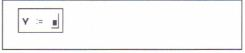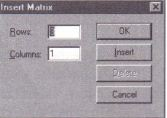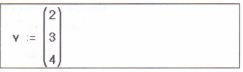# Computing with arrays MathCad Help

Variables can represent arrays as well as scalars. Defining a variable as an array is very much like defining a scalar. First type a variable name and a colon as you would with any other definition. Then create an array (vector or matrix) on the other s~depf the equation.

For example, to define a vector v, follow these step:

• Click in empty space and type v, followed  by the colon key (:)• Choose Matrix from the Insert menu to bring up a dialog box. Type 3 in the box next to “Rows” and 1in the box next to “Columns.”• Press “Create” and fill in the elementsYou can now use the name v in place of the actual vector in any equation. Figure 10- 1 demonstrates that the variable name v and the vector itself are interchangeable. Once you have defined a vector, you can of course define other vectors in terms of that vector, just as if you were doing mathematics on paper.

Do not use the same name for a scalar variable and a vector variable. This will simply redefine the variable.

Posted on November 20, 2015 in Vectors and Matrices